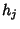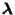## Kuhn-Tucker Theorem

A theorem in nonlinear programming which states that if a regularity condition holds andand the functionsare convex, then a solutionwhich satisfies the conditionsfor a Vector of multipliersis a Global Minimum. The Kuhn-Tucker theorem is a generalization of Lagrange Multipliers. Farkas's Lemma is key in proving this theorem.